The ratio $\frac{{C}_{p}}{{C}_{V}}=\gamma$ for a gas.  Its molecular weight is M. Its specification heat capacity at constant pressure is

(1) $\frac{R}{\gamma -1}$                (2) $\frac{\gamma R}{\gamma -1}$

(3) $\frac{\gamma R}{M\left(\gamma -1\right)}$         (4) $\frac{\gamma RM}{\left(\gamma -1\right)}$

Concept Questions :-

Specific heat
High Yielding Test Series + Question Bank - NEET 2020

Difficulty Level:

Which one of the following graph is correct at constant pressure

1.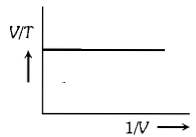2.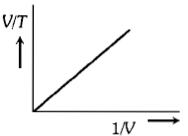3.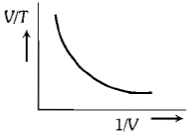4.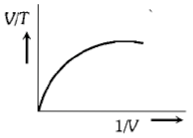Concept Questions :-

Ideal gas
High Yielding Test Series + Question Bank - NEET 2020

Difficulty Level:

The root-mean-square velocity of the molecules in a sample of helium is $\frac{5}{7}th$ of that of the molecules in a sample of hydrogen.  If the temperature of the hydrogen gas is 0$°$C, that of the helium sample is about:

(1) 0$°$C        (2) 4K         (3) 273$°$C         (4)100$°$C

Concept Questions :-

Types of velocity
High Yielding Test Series + Question Bank - NEET 2020

Difficulty Level:

Four molecules have speeds 2 km/sec, 3 km/sec, 4 km/sec and 5 km/sec.  The root mean square speed of these molecules (km/sec) is:

(1) $\sqrt{\frac{54}{4}}$          (2) $\sqrt{\frac{54}{2}}$          (3) 3.5            (4) 3$\sqrt{3}$

Concept Questions :-

Types of velocity
High Yielding Test Series + Question Bank - NEET 2020

Difficulty Level:

How many degrees of freedom the gas molecules have if, under STP, the gas density  and the velocity of sound propagation in it is 330 m${s}^{-1}$?

(1) 3       (2) 5           (3) 7              (4) 8

Concept Questions :-

Specific heat
High Yielding Test Series + Question Bank - NEET 2020

Difficulty Level:

The kinetic energy of one gram molecule of a gas at normal temperature and pressure is: (R = 8.31 J/mol-K)

[DPMT 1997; Pb. PMT 1997, 2000, 03; AFMC 1998; MH CET 1999]

(1) 0.56                (2)

(3)                   (4)

Concept Questions :-

Kinetic energy of gas
High Yielding Test Series + Question Bank - NEET 2020

Difficulty Level:

Gases exert pressure on the walls of containing vessel because  the gas molecules:

(1) Possess momentum

(2) collide with each other

(3) have finite volume

(4) obey gas laws

Concept Questions :-

Kinetic energy of gas
Maxwell distribution curve
High Yielding Test Series + Question Bank - NEET 2020

Difficulty Level:

The equation of state for 5 g of oxygen at a pressure P and temperature T, when occupying a volume V, will be: (where R is the constant)

(1) PV = 5RT

(2) PV = $\left(\frac{5}{2}\right)RT$

(3) PV = $\left(\frac{5}{16}\right)RT$

(4) PV = $\left(\frac{5}{32}\right)RT$

Concept Questions :-

Ideal gas
High Yielding Test Series + Question Bank - NEET 2020

Difficulty Level:

From the P - T graph, what conclusion can be drawn?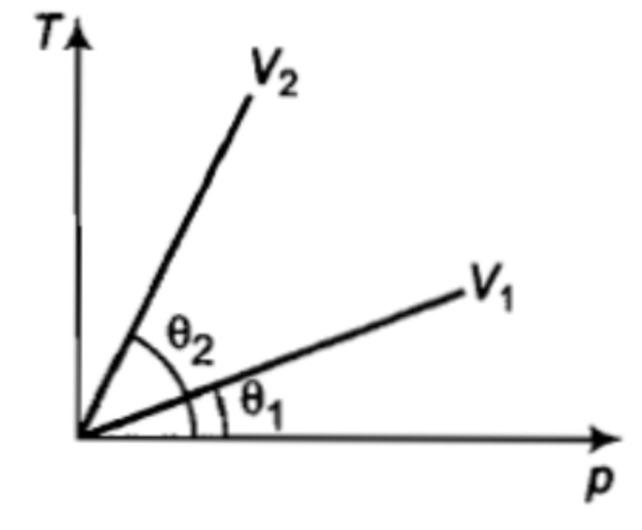(1) ${V}_{2}={V}_{1}$

(2) ${V}_{2}<{V}_{1}$

(3) ${V}_{2}>{V}_{1}$

(4) Nothing can be predicted

Concept Questions :-

Ideal gas
High Yielding Test Series + Question Bank - NEET 2020

Difficulty Level:

The root mean square speed of the molecules of an enclosed gas is V.  What will be the root mean square speed if the pressure is doubled, the temperature remaining the same?

(1) $\frac{v}{2}$      (2) v          (3) 2        (4) 4 v

Concept Questions :-

Types of velocity